#### Horizontal & Vertical Components of Vectors

A vector in space (3-dimensional) can be defined by its x, y, and z coordinates.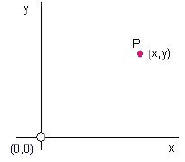Figure 1:
Point P in 2-D is defined by its Cartesian co-ordinates
(x , y) with respect to the origin (0,0)

We will analyze vectors in two dimensions.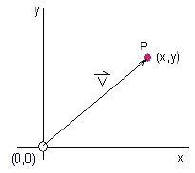Figure2:
The vector V maps the point P in terms
of its position from the origin

In a two dimensional frame we need only consider its x and y co-ordinates.Note: In figure 2, the tail of vector V is at the origin and its head ends at point P

Any vector in 2-D can be expressed in terms of its resultant.  A resultant is obtained by adding a vector's vertical component in the y direction (see figure 3) to its horizontal component in the x direction (see figure 4) .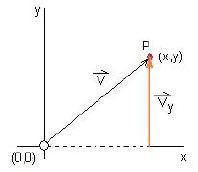Figure 3:
Vy is the vertical component of the
vector v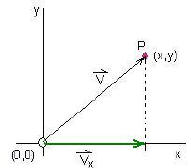Figure 4:
Vx  is the horizontal component of the
vector

Figure 5 is a diagram showing both the x and y components defining vector v.Vecor V is the resultant vector when Vx is added to Vy from the tail of Vx to the head of Vy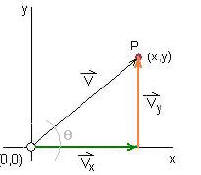Figure 5:
the x and y components of vector v

We can  define Vx and Vy in terms of  V and the angle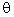using trigonometry:Know your basic trigonometry functions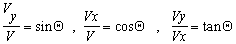Rearrange the first two equations to obtain expressions for Vy and Vx (the x and y components of vector v):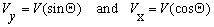The resultant vector v (see figure 5 above) will now be: = v+ vyExamples of problems involving vector components:

1. What are the horizontal and vertical components of an airplane moving at 300 km/h [E650N]?

Refer to diagram below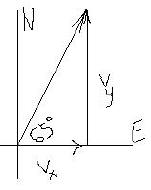Here=  65 0  and v = 300 km/h

Answer: Vx = 127 km [to the right] ; Vy = 272 km [up]Did you get the answer?Click here to see the solution or hint

2. What are the components of a vector 8 cm long with bearings [W150S]?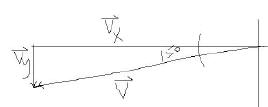Answer: Vx =7.7 cm [to the left] ; Vy = 2.1 cm[down]Did you get the answer?Click here to see the solution or hint